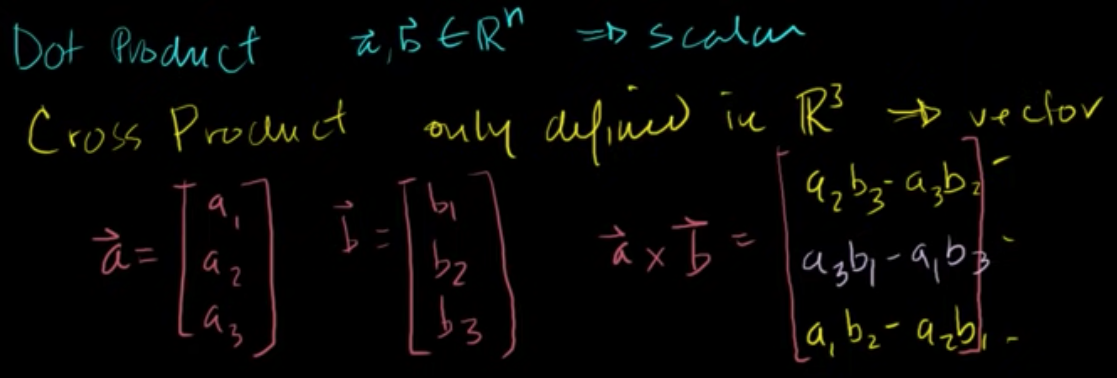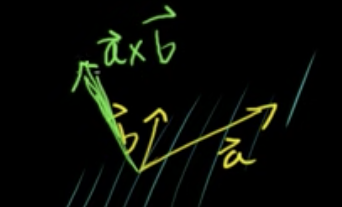## Cross product introductionThese are notes from Cross product introduction | Vectors and spaces | Linear Algebra | Khan Academy by Khan Academy.

The Cross Product is much more limited than Dot Product. Where the dot product is defined in any dimension ( $R_N$ ), the cross product is only defined in 3d ( $R_{3}$ ).

The dot product returns a scalar; the cross product a Vector.

Definition of the dot product:

$\vec{a} = \begin{bmatrix}a_1 \\ a_2 \\ a_3\end{bmatrix}, \vec{b} = \begin{bmatrix}b_1 \\ b_2 \\ b_3\end{bmatrix}$

$\vec{a} \times \vec{b} = \begin{bmatrix} a_2 \cdot b_3 - a_3 \cdot b_2 \\ a_3 \cdot b_1 - a_1 \cdot b_3 \\ a_1 \cdot b_2 - a_2 \cdot b_1 \end{bmatrix}$

• For first row in the returned vector, you ignore the top row and take $a_2 \cdot b_3 - a_3 \cdot b_2$
• For the 2nd row in the returned vector, you ignore the middle row of the vectors and take a similar product to the first; however, this time, you are doing it the opposite way around: $a_3 \cdot b_1 - a_1 \cdot b_3$
• For the 3rd row, you ignore the last row of input and make the same operation as the first row of the top 2 rows of input: $a_1 \cdot b_2 - a_2 \cdot b_1$.

The vector that's returned is orthogonal to both $\vec{a}$ and $\vec{b}$.Note that two vectors are orthogonal to those vectors. To find which direction it points in, you use the right-hand rule: take your right hand and put your index finger in the direction of $\vec{a}$ and your middle finger in the direction of $\vec{b}$, where your thumb is pointing in the direction of the returned vector.

What does orthogonal mean in this context? It means if $\vec{a} \cdot \vec{b} = 0$, the difference between orthogonal vectors and perpendicular vectors is orthogonal could also apply to 0 vectors.

You can prove it works by taking the dot product with one of the input vectors and the output vector:

$\begin{bmatrix} a_2 \cdot b_3 - a_3 \cdot b_2 \\ a_3 \cdot b_1 - a_1 \cdot b_3 \\ a_1 \cdot b_2 - a_2 \cdot b_1 \end{bmatrix} \cdot \begin{bmatrix}a_1 \\ a_2 \\ a_3\end{bmatrix}$

$= a_1 a_2 b_3 - a_1 a_3 b_2 + a_2 a_3 b_1 - a_2 a_1 b_3 + a_3 a_1 b_2 - a_3 a_2 b_1$

$= \mathbf{a_1 a_2 b_3} - a_1 a_3 b_2 + a_2 a_3 b_1 \mathbf{- a_2 a_1 b_3} + a_3 a_1 b_2 - a_3 a_2 b_1$

$= \mathbf{-a_1 a_3 b_2} + a_2 a_3 b_1 + \mathbf{a_3 a_1 b_2} - a_3 a_2 b_1$

$= \mathbf{a_2 a_3 b_1} \mathbf{- a_3 a_2 b_1}$

$= 0$